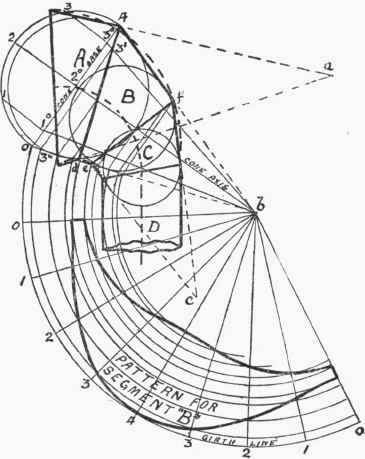An elevation of one form of this is shown in

Fig. 217, the head being made up in four pieces. So that each segment shall come out as a portion of a cone, it will be necessary to construct circles on the centre line, as shown by those described from centres n, o, and p. The shape of segment A is formed by drawing a cone, apex a, to touch the sphere whose centre is at n. The joint lines for the parts B, C, and D are determined by drawing a cone to touch spheres n and o; a cone, apex c, to touch spheres o and p, and the pipe, D, to touch the sphere p. Where the outside lines of the respective pairs of cones intersect will give the ends of the joint lines. Thus E F is the intersection of cones whose apexes are a and b, G H that of b and c, and J K the joint between cone c and the cylindrical pipe, D. To have a circular mouth, the line, L M, must be square to the cone axis, d a.Fig. 217.

The back and throat of a ventilator may be curved as shown by the dotted lines in Fig. 218, and the shape of the segments still obtained in the same manner as above.

The pattern for one segment only - that of B - is shown set out in Fig. 218. The complete cone is first constructed by making b 0 equal to b 4, and drawing in a base line, 0 4. Upon this base line a semicircle is drawn and divided into four parts. From each division point on the semicircle a perpendicular i s dropped on to the diameter, and then radial lines run on to the apex b. Where these radial lines intersect the joint lines, 4 d and f e, lines square to the cone axis are run on to the outside of the cone. Thus, all the construction lines required for the pattern are determined. The complete cone pattern is first marked out in the usual way by taking b 0 as radius and stepping eight lengths, each equal to one of the arcs on the semicircle along the girth line. These points are now joined up to b, and the radial lines so drawn cut off by using the lengths already found on the side b 0 of the cone. Thus, to follow the fixing of one pattern point, the line 3 3° is drawn on the semicircle, 3° joined to 6, and 3' 3" drawn parallel to the cone base, or square to the axis; the distance b 3" is then swung around on to the radial line, 6 3, and in this way a point on the pattern curve is obtained. In a similar manner the other points can be determined.Fig. 218.

The patterns for the segments A and C are not shown set out, as these, of course, can be developed in exactly the same way as explained above in connection with segment B. The pattern for the pipe D can be marked out as in an ordinary elbow, or similar to the straight pipe in Fig. 216.

A simple and cheap form of cowl can be designed by fitting a